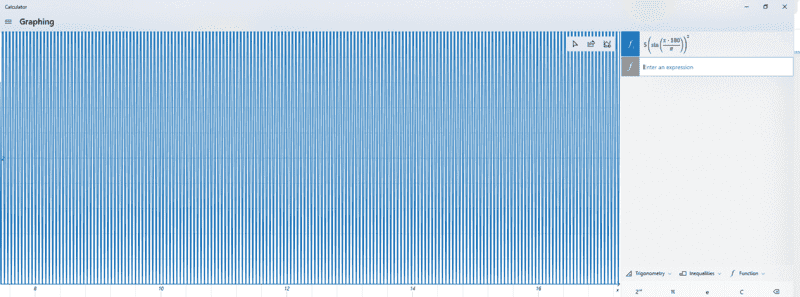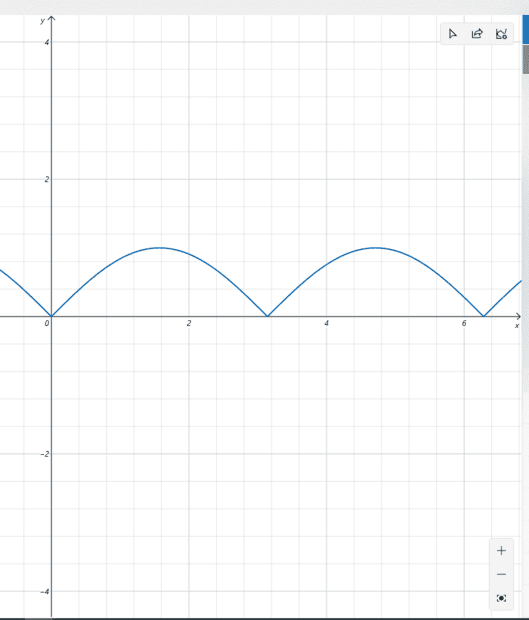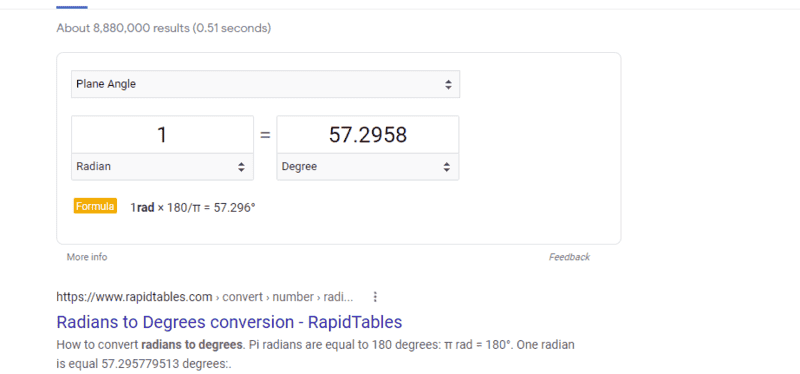# Equation to graph a sine wave that acts like a point on a unit circle

• I
btb4198
I need an equation to graph a sine wave that act like a unit circle but only positive numbers.
so I need it to be 0 at 0, A at 90 , 0 at 180, A at 270, 0 at 360, and A at 450 and so on and so on...

Now I know sin(0) is 0 in degrees and sin(90) 1
and I know if you Square a number is will always get you a positive number
so Y = A* sin(x)^2 should work, but x has to be in degrees, so you have to convert from radians to degrees.

with is 180/π

so now I have
Y = A * sin(x*(180/π) ^2
but this is not working on my computer's graphing Calculator:I tried doing adding - 90
Y = A * sin(x*(180/π -90) ^2

but that did not work
I never trying adding 90, - 360 and 360 nothing is working.
why is this ? what am I missing ?
ago the graph should be 0 on 0, 180 , and 360 and should be A( in this case 5) only on 90, 270 and 450 and so on and so on

Last edited by a moderator:

Staff Emeritus
Gold Member
Do you just want something like ##|sin(x)|##?

Also, your calculator probably assumes x is in radians to begin with, so when you try to convert it to degrees, you're shortening the period a lot more than you intended.

•btb4198
btb4198
Do you just want something like ##|sin(x)|##?

Also, your calculator probably assumes x is in radians to begin with, so when you try to convert it to degrees, you're shortening the period a lot more than you intended.
no that give me this:btb4198
I need it to go down on 90 and not 180

Staff Emeritus
Gold Member
Try multiplying by ##\pi/180## instead of ##180/\pi## inside of the sine function to get the right conversion to degrees.

btb4198
Try multiplying by ##\pi/180## instead of ##180/\pi## inside of the sine function to get the right conversion to degrees.
Thanks !
that work.is it wrong then?
how you were right!
my graph is working on

Homework Helper
2022 Award
I need an equation to graph a sine wave that act like a unit circle but only positive numbers.
so I need it to be 0 at 0, A at 90 , 0 at 180, A at 270, 0 at 360, and A at 450 and so on and so on...

Now I know sin(0) is 0 in degrees and sin(90) 1
and I know if you Square a number is will always get you a positive number
so Y = A* sin(x)^2 should work, but x has to be in degrees, so you have to convert from radians to degrees.

with is 180/π

so now I have
Y = A * sin(x*(180/π) ^2
but this is not working on my computer's graphing Calculator:
View attachment 290112

I tried doing adding - 90
Y = A * sin(x*(180/π -90) ^2

but that did not work
I never trying adding 90, - 360 and 360 nothing is working.
why is this ? what am I missing ?
ago the graph should be 0 on 0, 180 , and 360 and should be A( in this case 5) only on 90, 270 and 450 and so on and so on

From the basic trig identities \begin{align*} \cos^2 x+ \sin^2 x &= 1 \\ \cos^2 x - \sin^2 x &= \cos(2x) \end{align*} it follows that $\sin^2 x = (1 - \cos (2x))/2$. That's not what you want.

It sounds like what you want is $$y(x) = \frac{A}{2}\left(1 + \sin\left( \frac{\pi x}{180}\right)\right).$$

btb4198
From the basic trig identities \begin{align*} \cos^2 x+ \sin^2 x &= 1 \\ \cos^2 x - \sin^2 x &= \cos(2x) \end{align*} it follows that $\sin^2 x = (1 - \cos (2x))/2$. That's not what you want.

It sounds like what you want is $$y(x) = \frac{A}{2}\left(1 + \sin\left( \frac{\pi x}{180}\right)\right).$$
No
Office_Shredder was right...
I got the graph I wanted